• Размер: 807 Кб
• Количество слайдов: 10

## Описание презентации Problem 9. 32 What value of will по слайдам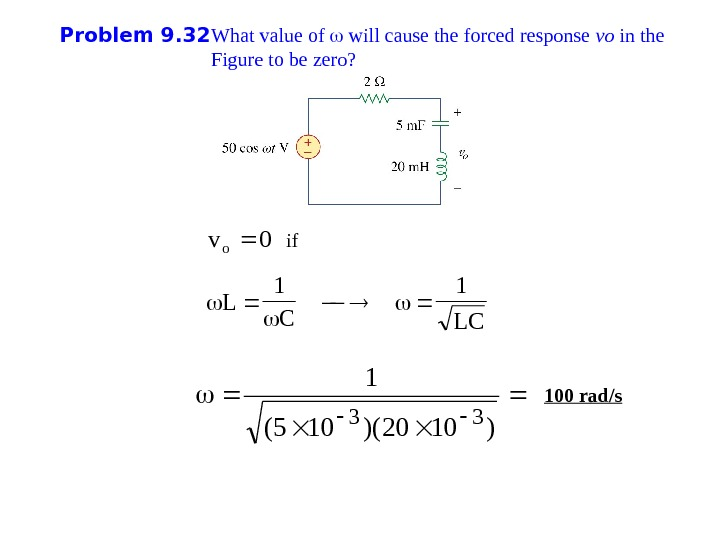Problem 9. 32 What value of will cause the forced response vo in the Fig ure to be zero? 0 vo LC 1 L )1020)(105( 1 33 if 100 rad/s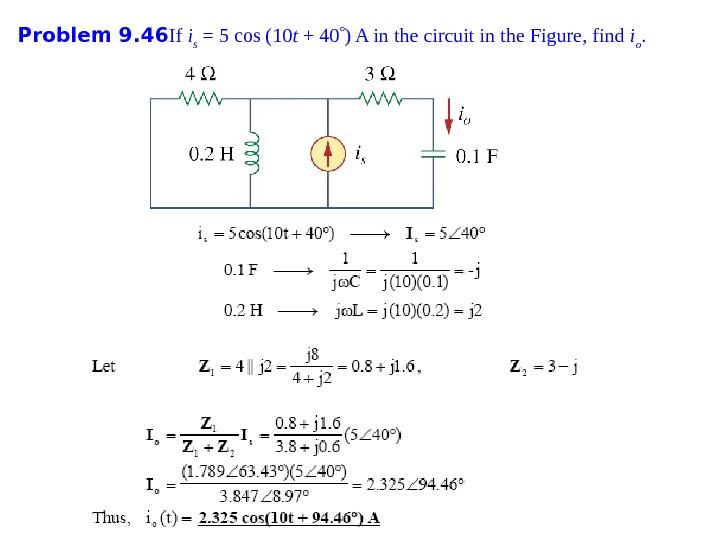Problem 9. 46 If i s = 5 cos (10 t + 40 ) A in the circuit in the Fig ure , find i o.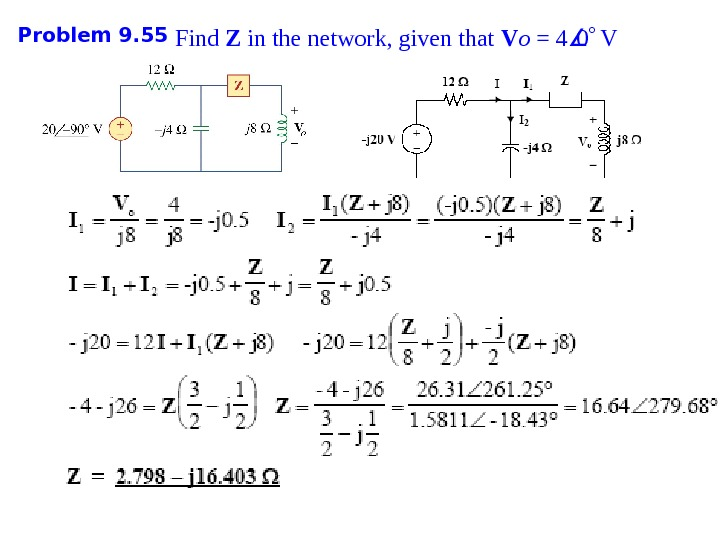Problem 9. 55 Find Z in the network, given that V o = 4 0 V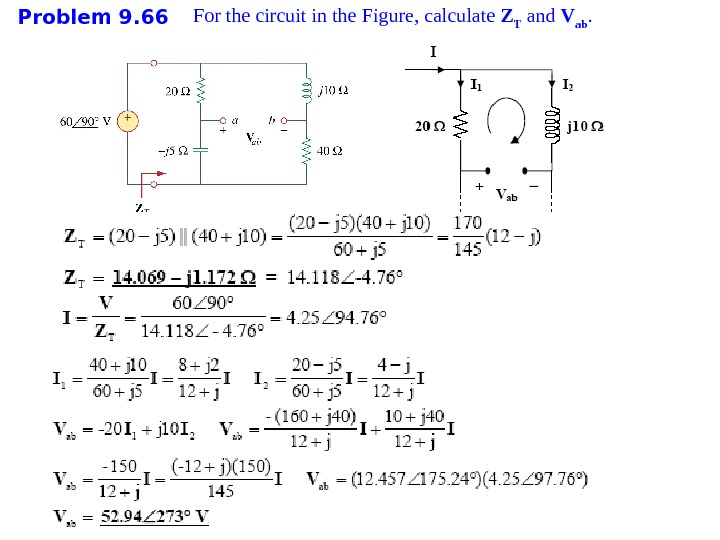Problem 9. 66 For the circuit in the Fig ure , calculate Z T and V ab.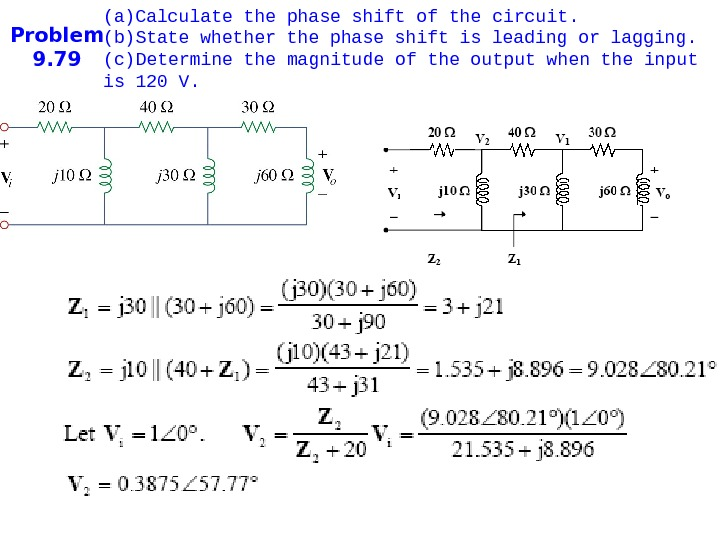Problem 9. 79 (a) Calculate the phase shift of the circuit. (b) State whether the phase shift is leading or lagging. (c) Determine the magnitude of the output when the input is 120 V.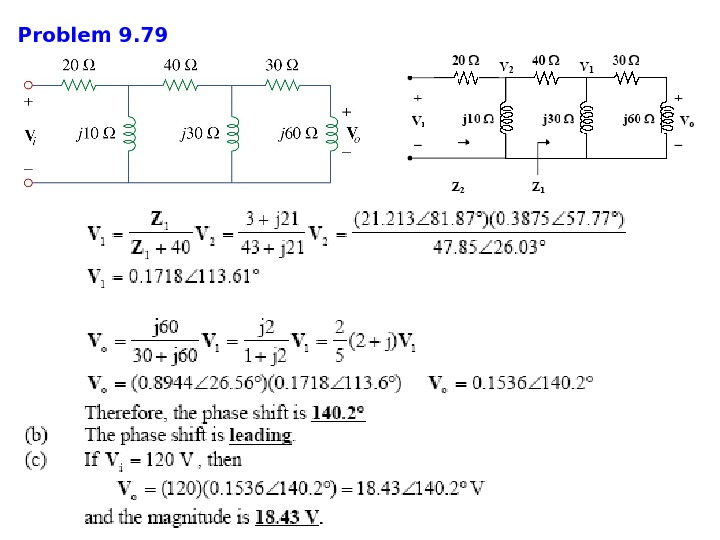Problem 9.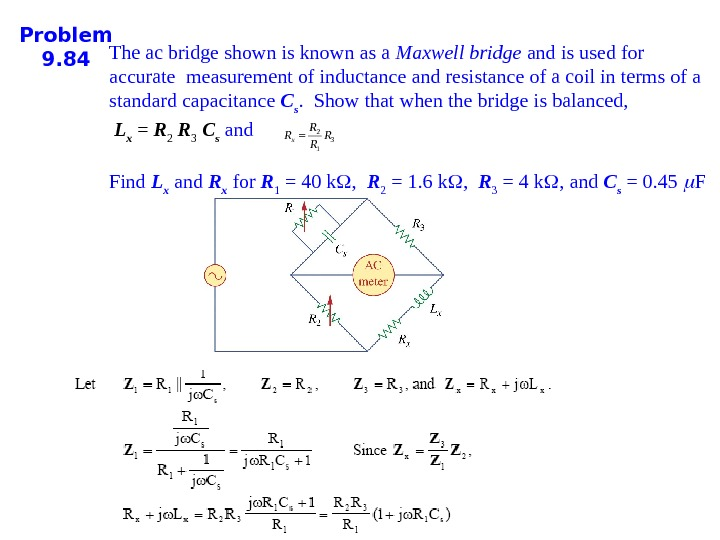Problem 9. 84 The ac bridge shown is known as a Maxwell bridge and is used for accurate measurement of inductance and resistance of a coil in terms of a standard capacitance C s. Show that when the bridge is balanced, L x = R 2 R 3 C s and Find L x and R x for R 1 = 40 k , R 2 = 1. 6 k , R 3 = 4 k , and C s = 0. 45 F 231 x RR RR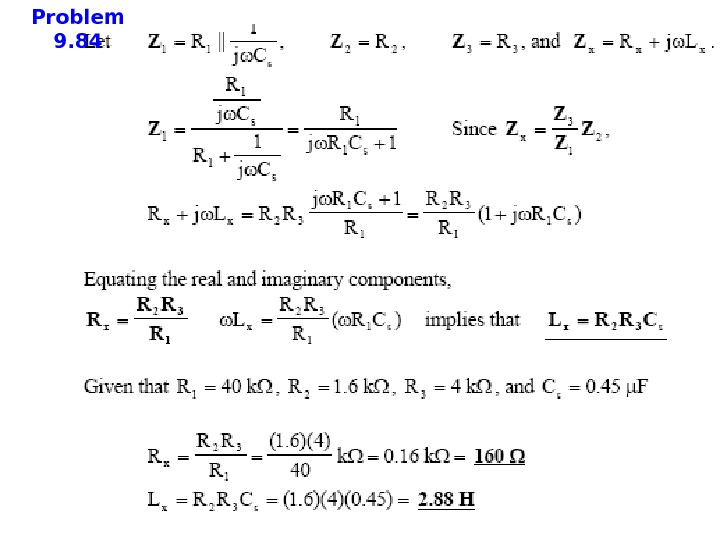Problem 9.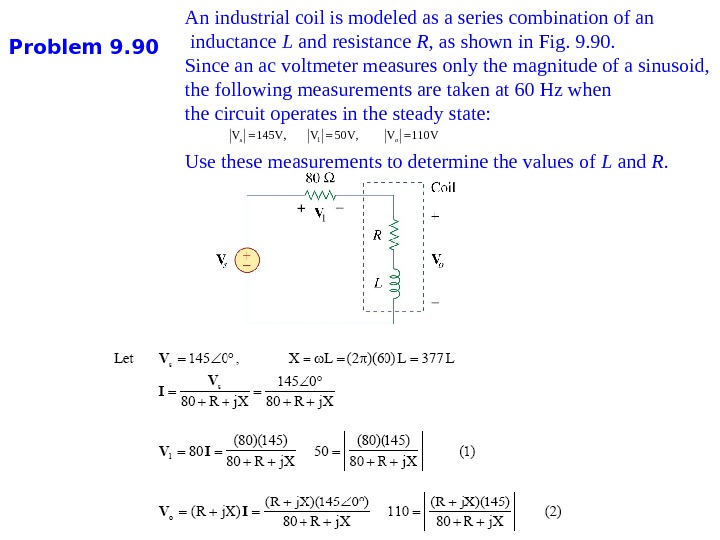Problem 9. 90 An industrial coil is modeled as a series combination of an inductance L and resistance R , as shown in Fig. 9. 90. Since an ac voltmeter measures only the magnitude of a sinusoid, the following measurements are taken at 60 Hz when the circuit operates in the steady state: Use these measurements to determine the values of L and R. 1 V 145 V, V 50 V, V 110 Vs o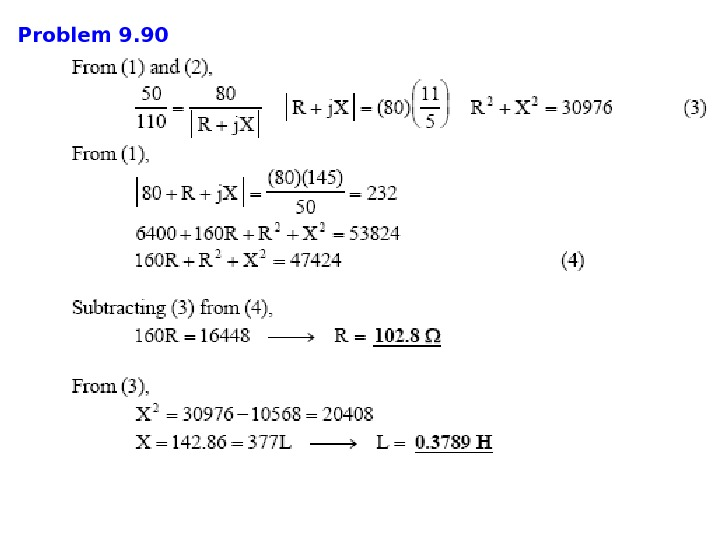Problem 9.